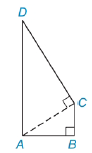Chapter 5.4, Problem 32EElementary Geometry For College St...

7th Edition
Alexander + 2 others
ISBN: 9781337614085

Solutions

Chapter
SectionElementary Geometry For College St...

7th Edition
Alexander + 2 others
ISBN: 9781337614085
Textbook Problem

In quadrilateral ABCD, B C ¯ ⊥ A B ¯ and D C ¯ ⊥ diagonal A C ¯ . If AB=4, BC= 3, and DC = 12, determine DA.To determine

To Find:

Length of DA.

Explanation

Given In quadrilateral ABCD, BC¯AB¯ and DC¯ diagonal AC¯. If AB=4, BC= 3, and DC = 12,

From the given data,

AB = 4, BC = 3 and DC =12.

From the figure,

BC¯AB¯ and DC¯ diagonalAC¯.

So ABC is a right triangle, then

From the Pythagorean Theorem,

AC2=AB2+BC2AC2=(4)

Still sussing out bartleby?

Check out a sample textbook solution.

See a sample solution

The Solution to Your Study Problems

Bartleby provides explanations to thousands of textbook problems written by our experts, many with advanced degrees!

Get Started

(939592)1/2

Applied Calculus for the Managerial, Life, and Social Sciences: A Brief Approach

Simplify: 112144

Elementary Technical Mathematics

Find f(a). f(x)=41x

Calculus (MindTap Course List)

Using for |x| < 1,

Study Guide for Stewart's Multivariable Calculus, 8th